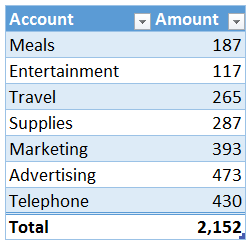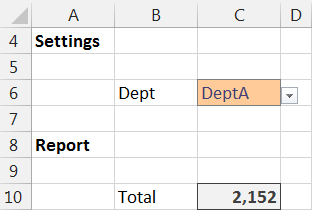## Referring to Tables Indirectly

In this post, we’ll allow the user to select a table name from a data validation drop-down and our Excel formulas will operate on the values from the selected table. Thanks to Neelima for asking about this technique!

## Objective

Before we get too far, let’s be clear about our objective. We have several data tables in several worksheets in our workbook. Each table stores data for one department. For example, here is the table for Department A, which has been named DeptA.We would like the user to pick a table name from a drop-down list, and we’d like our formula to provide the total for the selected department, as shown below.We’ll accomplish this with a little function known as INDIRECT. Let’s check it out now.

## INDIRECT

The INDIRECT function returns a valid Excel reference based on a text string. The syntax follows.

`=INDIRECT(ref_text, [a1])`

Where:

• ref_text is the text string of the desired Excel reference
• [a1] is an optional argument that allows us to specify an A1-style or RC-style reference

The INDIRECT function can create a valid Excel reference to a cell such as B10, to a range such as A1:B10, to a named reference, or to a table name such as DeptA. We have stored the data for our five departments in tables using the Insert > Table icon. We have named the tables DeptA, DeptB, DeptC, DeptD, and DeptE using the TableTools > Table Name ribbon field.

Our objective is to allow the user to enter the table name into a cell such as C6. Let’s say the user enters DeptA into cell C6. We can’t simply use the C6 reference in a function such as SUM(C6) to sum the values in the DeptA table. This is because the SUM function will return the sum of its argument C6, which in this case stores the text string value DeptA. The SUM function would thus return 0.

We need some way to tell the SUM function that we are not providing it with the sum range directly, but rather, we are providing it indirectly. That is, we are pointing it to the desired sum range. This is where the INDIRECT function comes to the rescue. It enables us to create a valid Excel reference from a text string, in this case, the text string of the table name stored in C6.

Rather than summing cell C6, we tell Excel to sum the values in the reference stored in C6 with the INDIRECT function, as follows.

`=SUM(INDIRECT(C6))`

The INDIRECT function converts the text string in C6, the table name DeptA, into a valid Excel reference. The SUM function sums the values stored in the DeptA table.

Now, the user can type the table name into cell C6 and the SUM function will return the total of all cells in the table. If we want to allow the user to select a table name from a list of tables, we can use the data validation feature to provide a drop-down.

## Data Validation

On the input cell, we set up data validation (Data > Data Validation) to allow a list. We simply enter the table names using a comma-separated list, as shown below.At this point, the user can select the desired department from the drop-down, and our formula updates accordingly, as shown below.But, what if we want to do something more elaborate than just simply sum the values? For example, what if we wanted to do a lookup…can we do that? Yes! The INDIRECT function simply returns a valid Excel reference and can be used in other functions, including the VLOOKUP function.

## VLOOKUP

If we wanted to allow the user to pick a department and we wanted our report to pull in the account values from the selected table, we could retrieve the values using a traditional lookup function such as VLOOKUP. The lookup range argument would simply use the INDIRECT function to provide the desired reference. For example, if we wanted to look up the value for the account name stored in B11 and the table name was stored in C6, we could use the following formula.

`=VLOOKUP(B11,INDIRECT(\$C\$6),2,0)`

Where:

• B11 is the lookup value, the account stored in B11
• INDIRECT(\$C\$6) is the lookup range, the table named in C6
• 2 will return the value from the 2nd column
• 0 means we want an exact matching value

The resulting workbook is shown below.The INDIRECT function returns a valid Excel range. We can use it to convert a text string, such as the table name DeptA, into a valid table reference that can be used in formulas and functions such as SUM and VLOOKUP. Thanks again Neelima for asking about this approach!

Posted in , ,### Jeff Lenning

I love sharing the things I've learned about Excel, and I built Excel University to help me do that. My motto is: Learn Excel. Work Faster.

### Want to learn Excel?

Our Campus Pass includes access to our entire Undergrad and Masters catalog. Gamification ensures it is the most fun you can have learning Excel :)

1.Zaigham on November 14, 2014 at 8:48 am

Easy to learn and nicely demonstrated.

•jefflenning on November 14, 2014 at 8:55 am

Thanks 🙂

2.Ricky on December 2, 2014 at 1:37 pm

Very nice! what if for example, I want to use a better name for the drop down list. So I don’t want to see DeptA, I want to see Department A, or A Department. is there a way to change just the drop down to refer to a table?

•jefflenning on December 2, 2014 at 2:08 pm

Ricky,

Thanks! To use a label in the drop-down other than the actual table name, you could set up a little mapping table and then use a lookup function such as VLOOKUP to make the translation. For example, a table could have a column for the friendly name used in the drop-down, and then another column that stores the actual table name. Then, your INDIRECT function would use a VLOOKUP to translate the selected label into the table name.

Hope this helps!

Thanks
Jeff

3.bahar on July 22, 2016 at 4:29 am

Hello,
I have a formula “=MAX(Table1[@[WISHLIST]:[MANUAL]]))” and i have this data in Table2 and Table3 too. Can you please advise the related INDIRECT formula i should use for dynamic table name? I could not do it.

•Kurt LeBlanc on July 25, 2016 at 12:26 pm

Hey Bahar

It took me a while but I figured it out:) I want to try explaining the logic I used…INDIRECT() returns a reference from text. When you wrap something in quotes, it is returned as text. You can put a cell reference, like in the blog, and join it with a text string wrapped in quotes with concatenation. Then you can wrap the whole thing with INDIRECT() to get a reference. The cell can be a drop-down list of the Table names, and the text string can be the “[@]wish list]:[manual]]” in your example. The @ symbol means “the data in the row your formula is in” and no symbol refers to the whole column so I’m not sure which you need, but I’ll write the formula below to make it clearer:

=MAX(INDIRECT(names&”[@[wish list]:[manual]]”))
OR
=MAX(INDIRECT(names&”[[wish list]:[manual]]”))

Let me know if this helps!
Kurt LeBlanc

4.bahar turkeli on July 27, 2016 at 7:02 am

Hello Kurt!
It works at Table1. But when I want to copy the formula and paste to Table2, it gives #REF error.

My main purpose is following: I want to copy a formula -let’s say =MAX([[WISHLIST]:[MANUAL]])) or =MAX(Table1[@[WISHLIST]:[MANUAL]]))- from Table1 and paste it to Table2. While I paste in Table 2, the formula turns out =MAX(Table1[[WISHLIST]:[MANUAL]])). However, what I want is to capture Table2 data. not Table1.

I hope it is clear enough. Any other suggestion welcomes.
Thank you!
Bahar

•Kurt LeBlanc on July 27, 2016 at 7:24 am

Hey Bahar

Where are you pasting the formula? Is it a new column in the table? If so, I don’t see a way to make the table name relative, but if you change the formula at he first row, the table will auto-fill the others:)

Kurt LeBlanc

5.bahar turkeli on July 28, 2016 at 4:33 am

Kurt, I am pasting it on another Table which is located on another sheet in the same workbook. Bahar.

•Kurt LeBlanc on July 28, 2016 at 7:12 am

Hey Bahar

I’m sorry, but I don’t see a way to do this exactly like you ere thinking. You can use the INDEX/MATCH functions to work around this though:)

Let me know if you need help setting it up.
Kurt LeBlanc

6.Robin Rascon on December 5, 2018 at 1:24 pm

Jeff: This is close but my dilemma is I’m working with a workbook that the department does not want to modify too much. However, I’d like to replace a formula that currently uses mutliple nested ifs, sumif and indirect to reference a sheet name from a cell. I think I might have problems with my solution because it’s only the last 5 digits of the cell using the RIGHT function.

I’m attempting to replace the formula with a much simpler SUMIFS function that pulls values from a table. The existing data is not currently in a table. I’m trying something like this:
=SUMIFS( tblCommtest[COMMS], tblCommtest[EID’#], ‘CA21′!C25,tblCommtest[Earnings Code],’CA21’!D25)
This works ok because I’m referencing the table directly. But the file has several worksheets with the same data but for different cost centers. These cost center sheets are referenced in column I of sheet CA21. Again, only the RIGHT 5 digits.

So, I tried to add the INDIRECT function to reference the table, but I am getting errors. Here’s an example:
SUMIFS( INDIRECT(“‘”&RIGHT(\$I25,5)&”‘!tblCommtest[COMMS],tblCommtest[EID’#],’CA21′!C25,tblCommtest[Earnings Code],’CA21’!D25)

Any suggestions? As always, thanks for all of your info!
Rob

7.Justin on February 4, 2019 at 8:48 am